Next: The Hall Effect Up: Magnetism Previous: The Lorentz Force

## Charged Particle in a Magnetic Field

Suppose that a particle of massmoves in a circular orbit of radius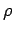with a constant speed. As is well-known, the acceleration of the particle is of magnitude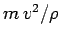, and is always directed towards the centre of the orbit. It follows that the acceleration is always perpendicular to the particle's instantaneous direction of motion.

We have seen that the force exerted on a charged particle by a magnetic field is always perpendicular to its instantaneous direction of motion. Does this mean that the field causes the particle to execute a circular orbit? Consider the case shown in Fig. 24. Suppose that a particle of positive charge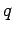and massmoves in a plane perpendicular to a uniform magnetic field. In the figure, the field points into the plane of the paper. Suppose that the particle moves, in an anti-clockwise manner, with constant speed(remember that the magnetic field cannot do work on the particle, so it cannot affect its speed), in a circular orbit of radius. The magnetic force acting on the particle is of magnitude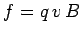and, according to Eq. (158), this force is always directed towards the centre of the orbit. Thus, if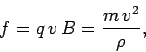(166)

then we have a self-consistent picture. It follows that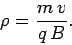(167)

The angular frequency of rotation of the particle (i.e., the number of radians the particle rotates through in one second) is(168)

Note that this frequency, which is known as the Larmor frequency, does not depend on the velocity of the particle. For a negatively charged particle, the picture is exactly the same as described above, except that the particle moves in a clockwise orbit.It is clear, from Eq. (168), that the angular frequency of gyration of a charged particle in a known magnetic field can be used to determine its charge to mass ratio. Furthermore, if the speed of the particle is known, then the radius of the orbit can also be used to determine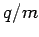, via Eq. (167). This method is employed in High Energy Physics to identify particles from photographs of the tracks which they leave in magnetized cloud chambers or bubble chambers. It is, of course, easy to differentiate positively charged particles from negatively charged ones using the direction of deflection of the particles in the magnetic field.

We have seen that a charged particle placed in a magnetic field executes a circular orbit in the plane perpendicular to the direction of the field. Is this the most general motion of a charged particle in a magnetic field? Not quite. We can also add an arbitrary drift along the direction of the magnetic field. This follows because the force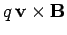acting on the particle only depends on the component of the particle's velocity which is perpendicular to the direction of magnetic field (the cross product of two parallel vectors is always zero because the anglethey subtend is zero). The combination of circular motion in the plane perpendicular to the magnetic field, and uniform motion along the direction of the field, gives rise to a spiral trajectory of a charged particle in a magnetic field, where the field forms the axis of the spiral--see Fig. 25.Next: The Hall Effect Up: Magnetism Previous: The Lorentz Force
Richard Fitzpatrick 2007-07-14